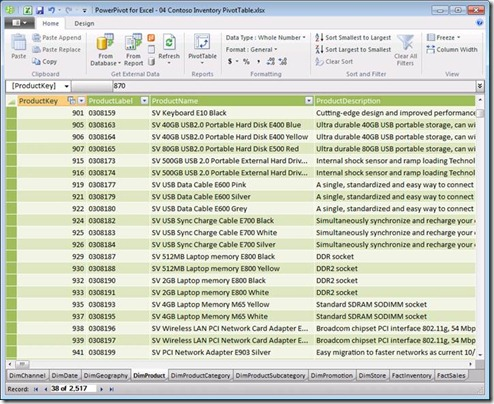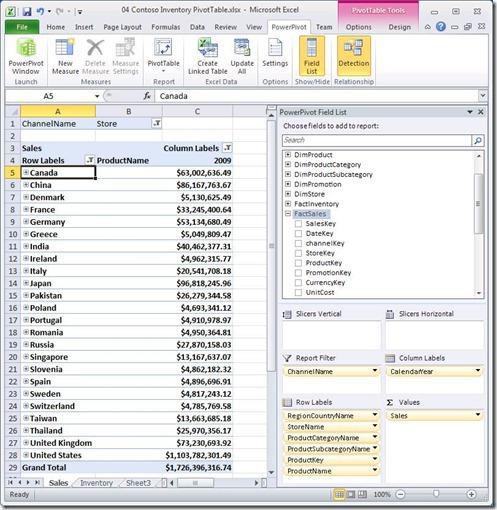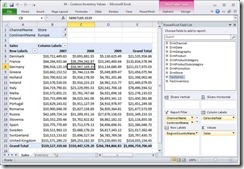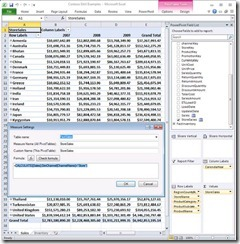Geeks With Blogs

# Intro

Data Analysis Expressions (DAX) In Excel 2010 PowerPivot

DAX: Excel data analysis functions to construct dynamic analysis custom pivots on front end – instead of SSAS MDX.

PowerPivot - building blocks to build BI solutions: import data tables from a various data sources, perform fast / custom DAX calculations on large in-memory datasets & display in PivotTables.

define calculations evaluated dynamically in different contexts

The ability to base a PivotTable on a set of multiple tables (as opposed to a single flattened table).

The ability to consume far larger data volumes (more rows) than can fit within an Excel worksheet.

Goal: Simplification of BI

• Ease-Of-Use - leverage standard Excel formula syntax (and some of Excel’s functions)
• Relational Data Simple relational data model: tables, columns, and relationships
• PivotTables - easier than MDX

DAX Online Function Reference: DAX Online Function Reference

Samples – Contoso Database– a Data Warehouse DB: http://www.microsoft.com/downloads/details.aspx?displaylang=en&FamilyID=868662dc-187a-4a85-b611-b7df7dc909fc millions of rows.

# PowerPivot

SSAS self-service BI for MS Office. consists of both a client-side component (PowerPivot for Excel) + server side component (PowerPivot for SharePoint).

PowerPivot for Excel - add-in for Excel 2010 http://powerpivot.com

PowerPivot for SharePoint add-in for SharePoint Excel Services as part of SQL Server 2008 R2 -for publishing and sharing PowerPivot workbooks.

The Excel client includes PowerPivot Window (an in-memory relational DB stored in a workbook - each table uses its own sheet) + PowerPivot Field List (PivotTable author - a task pane that is loaded in Excel as a replacement for Excel’s PivotTable field list)

PowerPivot Window:PowerPivot Field ListThe PowerPivot database is a set of tables that are loaded in memory and saved into an Excel workbook. When loaded in memory, these tables are viewed in the PowerPivot window, not on Excel’s worksheets.The tables are imported from a variety of external data sources - Relationships may be imported along with the data, or created within the PowerPivot window.

the DAX formula language can add calculated columns for tables and PivotTables measures

When the workbook is published to SharePoint the database is loaded into memory via Excel Services and exposed via PivotTable WebParts.

# DAX

#### Syntax

DAX syntax mimics Excel syntax - function composition , cell references.

· Operators and Constants - arithmetic operators, comparison operators , concatenation (&) , logical operators (||, &&). TRUE and FALSE as logical constants.

References to Columns name must be appear within square brackets, and it will optionally fully qualified ie preceded by the name of the table to which the column or measure belongs:

=SUM(Table1[Column2])

=[Measure2]/[Measure3]

measure names are globally unique across a DB, so don’t need to be fully qualified.

Unlike Excel formulas, DAX has no notion of addressing individual cells or ranges - DAX always refers to a column of data by providing the (qualified or unqualified) column name. When there is a row context, this reference to a column will be interpreted as the value of that column in the current row.

#### PowerPivot data types

DAX assumes that all values in columns (and the inputs and outputs of all DAX formulas) are one of the following six data types:

 Data Type (PowerPivot UI) Description Whole Number Integer Decimal Number Double precision real number Currency Four decimal places of precision (integer divided by 10,000) TRUE/FALSE Boolean Text String Date Datetime values beginning with Mar 1, 1900

This is slightly different from Excel, where everything is either a number (real) or a string. In Excel data types are handled by formatting numeric values rather than by using different data types. Note that Date values in DAX are restricted to dates after March 1, 1900.

DAX also has functions that return tables of data, but those tables may not be the final value in a measure or calculated column. Tables are used only as intermediate results, and are passed as arguments into additional functions, so that a DAX formula eventually returns a scalar value that has one of the six data types mentioned above.

#### Calculated Columns and Measures

· Calculated Columns - defined in the PowerPivot window by providing a column name and a DAX expression. Its values may be placed onto a PivotTable’s row labels / column labels / filter-slicer or used to define a measure - eg:

=[SalesAmount]-[TotalCost]

=[CityName]&", " & [StateProvinceName]

· Measures - named formulas defined in the PowerPivot Field List & added to the Values area of the PivotTable. Specify name & formula - evaluated separately for each cell in the Values area. Here are a couple of Measure definitions:

= SUM (FactSales[SalesAmount])

= AVERAGE (FactSales[SalesAmount])

· add measures to a PivotTable with a dimension on Row Labels.

· can easily build a measure that calculates the SUM, COUNT, MIN, MAX or AVERAGE of a column using checkboxes and dropdowns in the PowerPivot UI. can perform calculations that are far more powerful using DAX formulas instead of the five built in aggregations.

· Column and measure names must be unique in a DB

#### Functionality that doesn’t exist in Excel formulas

· relationships between tables, dynamic aggregation, context modification

· Schema functions:

• o Functions that navigate relationships between tables (more powerful than VLOOKUP)
• o Functions that take tables as arguments (aggregation over a table, filtering a table, etc.)
• o Functions that produce a table result (this result must then be an input to another function)

· Dynamic Measure Aggregation functions:

• o Functions that discover the current context for a calculation (e.g. has a specific filter been set?)
• o Functions that modify the context for a calculation (e.g. calculate formula for all products or years)
• o Functions that know about Time manipulation (e.g. parallel period, previous period, YTD, etc.)

#### Row Context and Filter Context

· in which each DAX expression is evaluated. the formula for a calculated column will be evaluated for each row of a table. a measure will be evaluated for each cell in the values area, each of which has its own “filter context” which is the combination of the cell’s row labels, column labels, report filters, and slicers.

· Row context is the current row - the same approach works in Excel. when there is a row context. When there is no row context, DAX cannot interpret the name of a column as a value. An expression will be evaluated using a row context for each row of the specified table.

· Filter Context - set of filters associated with one of the cells in the values area of a PivotTable.· This PivotTable has only one measure, with a single DAX formula defined as =SUM(FactSales[SalesAmount]) That single formula is being evaluated 72 distinct times, with 72 different results, and each of those 72 results has a distinct Filter Context. The context for each cell in the Values area includes the items on the row labels, the column labels, the report filter, (and slicers if they were being used.) The cell that is highlighted above has the following Filter Context.

• [RegionCountryName] = “Germany”
• [CalendarYear] = 2008
• [ChannelName]=”Store”
• [ContinentName]=”Europe”

· This can be thought of as applying four filters to the FactSales table prior to evaluating the formula which is simply the sum of the [SalesAmount] values for the rows that are left after applying those four filters.

· Relationships and Filter Context - relationships are always considered many-to-one. Applying a filter to a table on the “one” side of the relationship will also cause the table on the “many” side of the relationship to be filtered. Applying a filter to the table on the “many” side of a relationship will not have any impact on the table on the “one” side of the relationship.

· Measures and Filter Context - The formula that defines a measure is always evaluated multiple times – once for each cell in the Values area. And each of those evaluations has its own filter context. The presence of filter context for all measure evaluations (and the presence of row context for calculated columns,) is one of the reasons why you often cannot use the same formula to define a measure as you would use to define a calculated column. Generally, because a measure must be evaluated many times, including for any totals rows or columns, measure formulas must do some sort of aggregation.

# DAX Functions

#### BLANK() and Blank values

· Equivocates NULL values to 0, so that they can be included in aggregate calculations. However, if all values in an aggregated column are BLANK, the result will be BLANK. PivotTables automatically (by default) filter away any rows with blank results. Can compare the expression to BLANK() or use ISBLANK() function.

#### Functions from Excel

· DAX supports approximately eighty (80) functions from Excel.

· In places where the Excel function took a range (or a set of ranges,) the DAX function takes a column reference.

· Excel functions supported in DAX by Category:

· Date and Time: DATE , DATEVALUE, DAY , EDATE , EOMONTH , HOUR , MINUTE , MONTH, NOW , SECOND , TIME , TIMEVALUE , TODAY , WEEKDAY , WEEKNUM , YEAR , YEARFRAC

· Information: ISBLANK, ISERROR, ISLOGICAL, ISNONTEXT, ISNUMBER, ISTEXT

· Logical: AND, IF, IFERROR, NOT, OR, FALSE, TRUE,

· Math and Trig: ABS, CEILING, ISO.CEILING, EXP, FACT, FLOOR, INT, LN, LOG, LOG10, MOD, MROUND, PI, POWER, QUOTIENT, RAND, RANDBETWEEN, ROUND, ROUNDDOWN, ROUNDUP, SIGN, SQRT, SUM, SUMSQ, TRUNC

· Statistical: AVERAGE, AVERAGEA, COUNT, COUNTA, COUNTBLANK, MAX, MAXA, MIN, MINA

· Text: CONCATENATE, EXACT, FIND, FIXED, LEFT, LEN, LOWER, MID, REPLACE, REPT, RIGHT, SEARCH, SUBSTITUTE, TRIM, UPPER, VALUE

#### FORMAT (Value, Format_text)

· Instead of Excel’s TEXT function, DAX has a FORMAT function for converting various numeric and date values to strings.

=FORMAT([TestDate],"mmmm dd yyyy hh:mm:ss")

#### Functions to aggregate expressions

· DAX implements aggregation functions from Excel including SUM, AVERAGE, MIN, MAX, COUNT against a reference to a column or SUMX, AVERAGEX, MINX, MAXX, COUNTX functions over the rows of a table.

=SUM ( FactSales [SalesQuantity])

=SUMX (FactSales, [SalesQuantity])

=SUMX (FactSales, FactSales[SalesQuantity])

· usefule in measures because the context in which they are evaluated will change (filter) the table over which the aggregation is being performed.

#### COUNTROWS (Table)

· COUNTROWS is similar to the other COUNT functions (COUNT, COUNTA, COUNTX, COUNTAX, COUNTBLANK) and returns the count of rows in that table..

#### RELATED (Column) and RELATEDTABLE (Table)

· for following relationships and retrieving related data from another table - more powerful than Excel VLOOKUP (which only returns the first match).

· RELATED (Column) follows existing many-to-one relationship(s) from the many side to the one side and returns the single matching value from the other table.

=RELATED(DimStore[StoreName])

· RELATEDTABLE (Table) follows a relationship in either direction (many-to-one or one-to-many) and returns a table containing all the rows that are related to the current row from the specified table. This function returns a table and not a scalar value à an intermediate result that can be input into an aggregation function.

= SUMX(RELATEDTABLE(FactSales), FactSales[SalesAmount])

#### FILTER(Table, Condition) and DISTINCT (Column) Table Functions

· FILTER (Table, Condition) returns a subset of the specified table rows where the condition is true.

=SUMX( FILTER (FactSales,RELATED(DimGeography[CityName])="Baltimore") , [SalesAmount])

· DISTINCT (Column) returns a table containing the unique values within the specified column.

#### CALCULATE(Expression, SetFilter1, SetFilter2,...)

· allows any DAX expression to be evaluated in a specified filter context. This is equivalent to defining a measure and also defining a set of filters that will be used to provide a context in which the measure is to be evaluated. does the following:

o Using the SetFilter arguments, modify the Filter Context

o If there is a Row Context, move that Row Context onto the Filter Context

o Evaluate the Expression in the newly modified Filter Context· Syntax :

CALCULATE (expression [,SetFilter1] [,SetFilter2]...)

· eg

= CALCULATE ([Sales], DimChannel [ChannelName]=”Store”)

· A SetFilter argument in CALCULATE may be either of the following:

o A Boolean expression that refers to a single column such as DimChannel [ChannelName] =”Store” - Note that the Boolean expression may not refer to measures and may not invoke the Calculate function nor any table scanning functions including aggregation functions or table valued functions.

o A table expression such as Filter(Table1, Condition)

#### VALUES(column)

· return all the valid values for that column in the current filter context. The result comes back as a table of values, even though the table may have only one value (or even no values). This function is an easy way to ask about the current filter context. This function will often be used within an IF function:

= IF (COUNTROWS(VALUES(DimGeography[RegionCountryName]))=1,

VALUES(DimGeography[RegionCountryName]),

"No single country selected")

#### CALCULATETABLE(TableExpression, SetFilter1, SetFilter2,...)

· CALCULATETABLE is just like CALCULATE, except that the expression and the result are both tables - use to change the filter context and then construct a table in that new context.

#### ALL(Table) and ALL(Column1 [,Column2]...)

· table valued function that that causes the filter context for the specified columns to be ignored. useful when used as a table valued SetFilter argument within the CALCULATE function.

= [Sales]/CALCULATE([Sales], ALL(DimProduct))

#### ALLEXCEPT (Table [,Column1] [,Column2]...)

· a shorthand notation for a series of ALL functions.

# Time Intelligence Functions

· Compare some number to a comparable number for a different time period. Best practices - create a time dimension table in PowerPivot data that contains one row for every date that might exist in the data. DAX uses this table to construct a set of dates for each calculation. The only column that matters is the date column itself - others aren’t needed by DAX, just a reference to build PivotTables.

· calculate a set of dates as a table – use as a SetFilter argument in the CALCULATE function. Can be a single date, a set of contiguous dates, a set of non-contiguous dates, or dates that correspond to a calendar month or quarter or year. Can then use DAX functions to shift an arbitrary interval forward or backward in time

#### Functions that return a single date

• FIRSTDATE (Date_Column)
• LASTDATE (Date_Column)
• FIRSTNONBLANK (Date_Column, Expression)
• LASTNONBLANK (Date_Column, Expression)
• STARTOFMONTH (Date_Column)
• STARTOFQUARTER (Date_Column)
• STARTOFYEAR (Date_Column [,YE_Date])
• ENDOFMONTH (Date_Column)
• ENDOFQUARTER (Date_Column)
• ENDOFYEAR (Date_Column [,YE_Date])

#### Functions that return a table of dates

• PREVIOUSDAY (Date_Column)
• PREVIOUSMONTH (Date_Column)
• PREVIOUSQUARTER (Date_Column)
• PREVIOUSYEAR (Date_Column [,YE_Date])
• NEXTDAY (Date_Column)
• NEXTMONTH (Date_Column)
• NEXTQUARTER (Date_Column)
• NEXTYEAR (Date_Column [,YE_Date])
• DATESMTD (Date_Column) - calculate the set of dates in the period
• DATESQTD (Date_Column)
• DATESYTD (Date_Column [,YE_Date])
• SAMEPERIODLASTYEAR (Date_Column)
• DATEADD (Date_Column, Number_of_Intervals, Interval) - shift
• DATESBETWEEN (Date_Column, Start_Date, End_Date)
• DATESINPERIOD (Date_Column, Start_Date, Number_of_Intervals, Interval)
• PARALLELPERIOD (Date_Column, Number_of_Intervals, Interval)

#### Functions that evaluate expressions over a time period

• TOTALMTD (Expression, Date_Column [, SetFilter])
• TOTALQTD (Expression, Date_Column [, SetFilter])
• TOTALYTD (Expression, Date_Column [, SetFilter] [,YE_Date])
• OPENINGBALANCEMONTH (Expression, Date_Column [,SetFilter]) - based on the last date of the previous period
• OPENINGBALANCEQUARTER Quarter (Expression, Date_Column [,SetFilter])
• OPENINGBALANCEYEAR (Expression, Date_Column [,SetFilter] [,YE_Date])
• CLOSINGBALANCEMONTH (Expression, Date_Column [,SetFilter]) - based on the last date of the current period
• CLOSINGBALANCEQUARTER (Expression, Date_Column [,SetFilter])
• CLOSINGBALANCEYEAR (Expression, Date_Column [,SetFilter] [,YE_Date])

Comments on this post: Data Analysis Expressions (DAX)

# calculated column current MonthHi, strong blog, nice!
Q: How create calculated 'Date' column where the
value=1 when selected date is current month
value=0 when selected date is not current month
Goal: after moving 'Date'.[CurrentMonth] on the Filter area and selecting '1' then show measures only for current month.
thanks
Regards
Left by blase on Apr 17, 2016 11:43 AM

# re: Data Analysis Expressions (DAX)There's a lot to learn about all these functions. - Paradise Home Improvement Charlotte
Left by Mike Abbott on Jan 06, 2017 9:11 PM